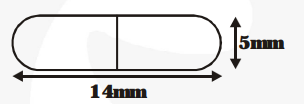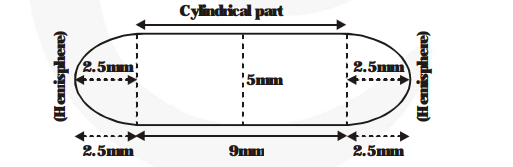# A medicine capsule is in the shape of a cylinder with two hemispheres struck to each of its ends (see figure).

Question:

A medicine capsule is in the shape of a cylinder with two hemispheres struck to each of its ends (see figure). The length of the entire capsule is 14 mm and the diameter of the capsule is 5 mm. Find its surface area.Solution:

Surface area of the cylindrical part $=2 \pi \times \mathrm{r} \times \mathrm{h}$$=2 \pi \times\left(\frac{\mathbf{5}}{\mathbf{2}}\right) \times 9 \mathrm{~mm}^{2}=45 \pi \mathrm{mm}^{2}$

Sum of the curved surface areas of two hemispherical parts.

$=2\left\{\mathbf{2 \pi} \times\left(\frac{\mathbf{5}}{\mathbf{2}}\right)^{2}\right\} \mathbf{m m}^{2}=25 \pi \mathrm{mm}^{2}$

Total surface area of the capsule

$=45 \pi+25 \pi \mathrm{mm}^{2}=70 \pi \mathrm{mm}^{2}$

$=70 \times \frac{\mathbf{2 2}}{\mathbf{7}} \mathrm{mm}^{2}=220 \mathrm{~mm}^{2}$